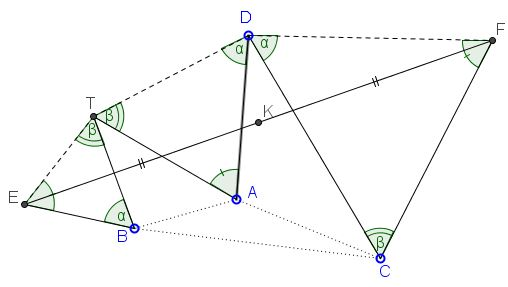# Bottema with Similar Triangles

### Problem

Assume triangles $BET,$ $DAT,$ and $DFC$ are similar as shown below.Prove that $K,$ the midpoint of $EF$ is independent of the position of $D.$

### SolutionAssuming the point names as complex numbers, the similarity of the three triangles could be expressed as

$\displaystyle\frac{T-E}{B-E}=\frac{T-A}{D-A}=\frac{C-F}{D-F}=u,$

for a complex number $u.$ From here,

$\displaystyle\frac{(T+C)-(E+F)}{(B+D)-(E+F)}=u,$

implying

$\displaystyle\frac{(A+C)-(E+F)}{(A+B)-(E+F)}=u,$

which exactly means that $E+F$ and, with it, $\displaystyle\frac{E+F}{2}$ is independent of $D.$

### Acknowledgment

This problem is a follow-up of the one posted by Dao Thanh Oai (Vietnam) at the CutTheKnotMath facebook page. The simple solution above has been posted by Leo Giugiuc (Romania) who also observed that Dao's problem extends to the one above. Here's Dao's formulation:

Triangles $BET,$ $TAD,$ $DFC$ are similar isosceles. Prove that the midpoint $K$ of $EF$ is independent of the position of $D.$### Bottema's Theorem